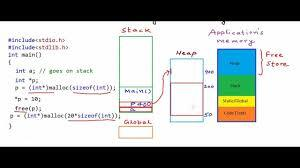# 手把手透析 C 语言堆内存申请 malloc 及扩容 realloc## 官方文档

• Following is the declaration for malloc() function.

void *malloc(size_t size)

• Following is the declaration for realloc() function.

void *realloc(void *ptr, size_t size)

## 实测与透析

• new_size 比 size 小的情况测试

 #include<stdio.h> #include<stdlib.h> void main(){    char *p = (char *)malloc(10 * sizeof(char));//p point 10 size blocks;    *(p+0) = 65;    *(p+1) = 66;    char *temp_p = NULL;    temp_p =(char *) realloc(p,2);//因为2比p的原始大小10还小，所以这里会将p的大小缩小为2，相当于temp_p和p指向的还是p指的那同一块内存空间，只是size缩小为2了。    *(temp_p +3) = 'Z';    printf("The value of *p: %c\n",*p );//这个时p还在；    printf("The value of *temp_p: %c\n",*(temp_p));//有值，即*p    printf("The value of *(temp_p +1): %c\n",*(temp_p +1));//有值，即*(p + 1)    printf("The value of *(temp_p +2): %c\n",*(temp_p +2));//没有值，因为没有被赋值过值    printf("The value of *(temp_p +3): %c\n",*(temp_p +3));    //此处有值，是'Z'，因为手动赋值过，但具体存的位置是不是p被裁剪前的*(p + 3)，这个很难说.}

• new_size 比 size 大，但是 p 后面还有富余

p 后面还有空闲的 memory block 可供延续，这个是不会自动释放 p 的

#include<stdio.h>#include<stdlib.h>void main(){    char *p = (char *)malloc(10 * sizeof(char));//p point 10 size blocks;    *(p+0) = 65;    *(p+1) = 66;    *(p+2) = 67;    *(p+3) = 68;    char *temp_p = NULL;    temp_p =(char *) realloc(p,20);    printf("The value of temp_p:\n %c\n",*(temp_p));    printf("The value of *(temp_p +1):\n %c\n",*(temp_p +1));    printf("The value of *(temp_p +2):\n %c\n",*(temp_p +2));    printf("The value of *(temp_p +3):\n %c\n",*(temp_p +3));}//证据->可以在中途free(p)试试//(加入free(p)，这种情况会导致temp_p也失效)，很容易对比分析：#include<stdio.h>#include<stdlib.h>void main(){    char *p = (char *)malloc(10 * sizeof(char));//p point 10 size blocks;    *(p+0) = 65;    *(p+1) = 66;    *(p+2) = 67;    *(p+3) = 68;    char *temp_p = NULL;    temp_p =(char *) realloc(p,20);    if(temp_p){        free(p);    }    printf("The value of temp_p:\n %c\n",*(temp_p));    printf("The value of *(temp_p +1):\n %c\n",*(temp_p +1));    printf("The value of *(temp_p +2):\n %c\n",*(temp_p +2));    printf("The value of *(temp_p +3):\n %c\n",*(temp_p +3));}

• new_size 的值太大了，p 后面已经没有什么富余了

#include<stdio.h>#include<stdlib.h>void main(){    char *p = (char *)malloc(10 * sizeof(char));//p point 10 size blocks;    *(p+0) = 65;    *(p+1) = 66;    *(p+2) = 67;    *(p+3) = 68;    char *temp_p = NULL;    temp_p =(char *) realloc(p,20000000);    printf("The value of *p:\n %c\n\n\n",*p);    printf("The value of *temp_p:\n %c\n",*(temp_p));    printf("The value of *(temp_p +1):\n %c\n",*(temp_p +1));    printf("The value of *(temp_p +2):\n %c\n",*(temp_p +2));    printf("The value of *(temp_p +3):\n %c\n",*(temp_p +3));}/*new_size 的值太大了，p后面已经没有什么富余了。   这个时候realloc的行为是：重新找一块地儿，并且将p中存储的原值拷贝过去并自动释放p,证据：第20行，打印p的第一个元素时，已经打印不出来了，因为已经被free了。但是后面打印*temp_p 和 *(temp_p +1)  *(temp_p +2) *(temp_p +3) 时，均可以正常打印！*/## 评论